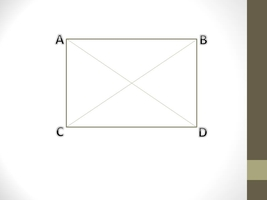# A rectangular pen for a pet is 5 feet longer than it is wide. Give the possible width of the pen...

## Question:

A rectangular pen for a pet is 5 feet longer than it is wide. Give the possible width of the pen if its area must be 204 and 750 square feet, inclusively.

## Rectangular Shapes

A rectangle is a type of quadrilateral that has a pair of parallel sides in which opposite sides are parallel and equal in length.

Interior angles of a rectangle are equal and of {eq}90^{\circ}. {/eq}

A rectangle has two diagonals that have the same length.#### Perimeter of a Rectangle

{eq}\displaystyle P = 2 \times (l+w) {/eq}

here {eq}P = {/eq} perimeter of the rectangle

{eq}l = {/eq} length

{eq}w = {/eq} width of the rectangle

#### Area of a Rectangle

{eq}\displaystyle A = l \times w {/eq}

here

{eq}A = {/eq} area

{eq}l = {/eq} length

{eq}w = {/eq} width of the rectangle

Given that -

A rectangular pen for a pet is 5 feet longer than its wide.

so

{eq}\displaystyle l = w+5 ---------(1) {/eq}

When the area of the pen is 204 square feet

{eq}\displaystyle 204 = l \times w {/eq}

from equation(1)-

{eq}\displaystyle 204 = (w+5)w {/eq}

{eq}\displaystyle 204 = w^{2}+5w {/eq}

{eq}\displaystyle w^{2}+5w-204 = 0 {/eq}

{eq}\displaystyle w = \frac{-5 \pm \sqrt{5^{2}-4 \times 1 \times (-204) }}{2 \times 1} {/eq}

{eq}\displaystyle w = \frac{-5 \pm \sqrt{841 }}{2} {/eq}

{eq}\displaystyle w = \frac{-5 \pm 29}{2} {/eq}

So {eq}\displaystyle w = \frac{-5 + 29}{2} = 12 {/eq}

and

{eq}\displaystyle w = \frac{-5 - 29}{2} = -17 {/eq}

{eq}\displaystyle w = 12 feet {/eq} because the width of a rectangle can not be negative So {eq}w =-17 {/eq} is unacceptable.

Now when the area is 750 square feet.

{eq}\displaystyle 750 = (w'+5)w' {/eq}

{eq}\displaystyle w'^{2}+5w'-750 = 0 {/eq}

{eq}\displaystyle w' = \frac{-5 \pm \sqrt{5^{2}-4 \times 1 \times (-750)}}{2 \times 1} {/eq}

{eq}\displaystyle w' = \frac{-5 \pm \sqrt{25+3000}}{2} {/eq}

{eq}\displaystyle w' = \frac{-5 \pm \sqrt{3025}}{2} {/eq}

{eq}\displaystyle w' = \frac{-5 \pm 55}{2} {/eq}

So

{eq}\displaystyle w' = \frac{-5 + 55}{2} = 25 {/eq}

and

{eq}\displaystyle w' = \frac{-5 - 55}{2} = -30 {/eq}

{eq}\displaystyle w'=25 {/eq} because the width of a rectangle can not be negative So {eq}w' =-30 {/eq} is unacceptable.